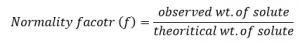# Normality Factor (f)

Normality factor is defined as the ratio of observed wt. of solute to the theoretical wt. of the solute required to prepare a solution of desired normality.Let us prepare a decinormal solution of Na2CO3 100ml volumetric flask.
We have,
1000 ml of 1N Na2CO3 solution ≡ 1 g. eqv. of Na2CO3 ≡ 53 g. of Na2CO3
Then,
100 ml of 0.1N Na2CO3 solution = 53/1000 ×100 ×0.1g of Na2CO3=0.53g
Observed wt. of Na2CO3 = 0.583g
0.583g of Na2CO3 is transferred into a clean volumetric flask and it is dissolved in water to make exactly 100ml in the volumetric flask.
f=0.583g/0.53g=1.1
Here the concentration of the prepared Na2CO3 is
N/10 (f=1.1)=0.11 N
The prepared solution has concentration slightly greater than decinormal. In order to prepare exactly decinormal Na2CO3 solution from the prepared solution water is required to add on the basis of the principal of dilution.
The principle states that, “the product of volume and concentration factor of the solution at any dilution is constant provided mass of the solution is constant.”
It means,
V1 ml of S1N solution of A = 100 ml of N/10 (f=1.1) Na2CO3 solution
Or, V x N/10 = 100 x N/10 x 1.1